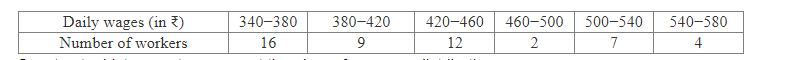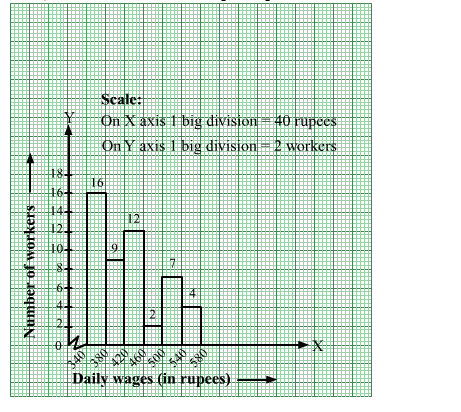# The daily wages of 50 workers in a factory are given below:Question:

The daily wages of 50 workers in a factory are given below:Construct a histogram to represent the above frequency distribution.

Solution:

The given frequency distribution is in exclusive form.
We will represent the class intervals [daily wages (in rupees)] along the x-axis & the corresponding frequencies [number of workers] along the y-axis.
The scale is as follows:
On x-axis: 1 big division = 40 rupees
On y-axis: 1 big division = 2 workers
Because the scale on the x-axis starts at 340, a kink, i.e., a  break, is indicated near the origin to signify that the graph is drawn with a scale beginning at 340
and not at the origin.
We will construct rectangles with the class intervals as bases and the corresponding frequencies as heights.
Thus, we will obtain the following histogram: